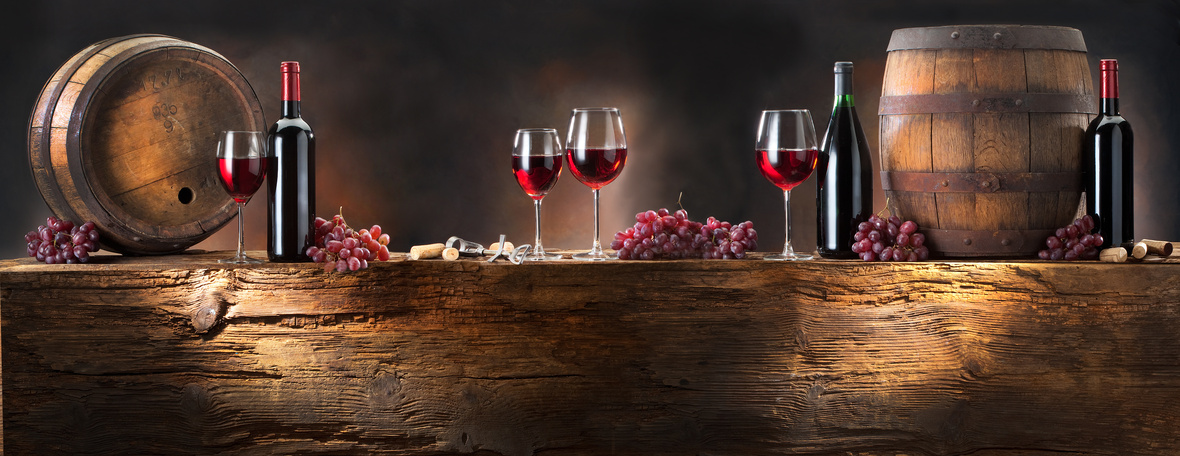# Renew, Reward, Recharge!

 /* styles */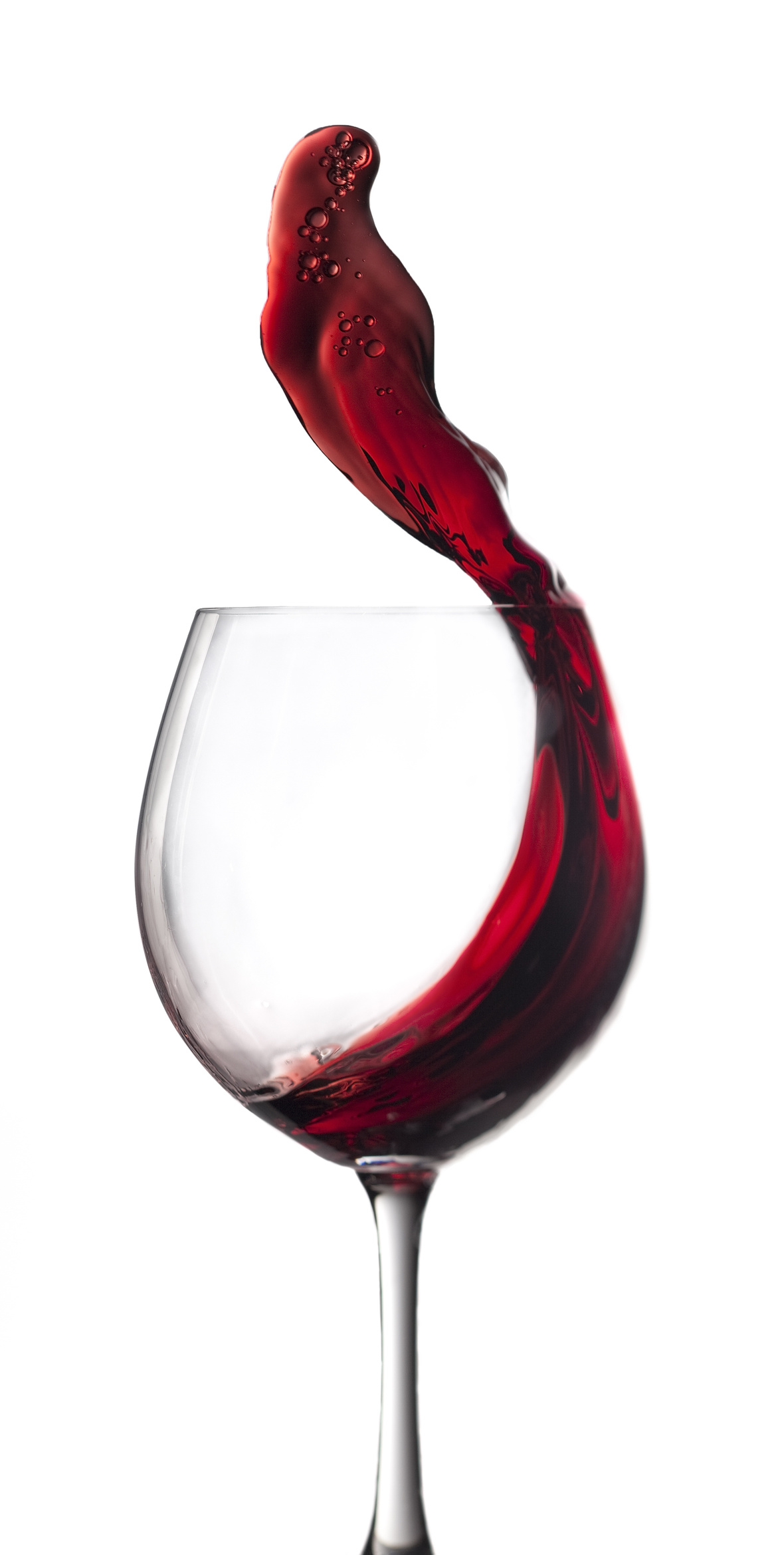Hi Wine & Spirits Lover,

The Shall We Wine team is excited to share our monthly newsletter. Our goal is to introduce you to our favorite selections, producers, distillers, varietals, regions and places to purchase and drink fabulous wine and spirits!

Cheers,

The Shall We Wine Team

 /* styles */We ended 2020 with a cheers to a New Year of Wine, Wellness and Wealth ✰✰✰✰

This 2021, let's continue to strengthen the community bond of the Shall We Wine tribe and build around matters of opportunity, learning and pleasure!

Shall We Wine is here to guide you. We're here to help you put your snazzy wine & spirits feet forward! From a range of SWW Memberships, wine & spirits style features, taste preference coverage and at an array of price points, it is a year of renewed optimism. Reward yourself.

Out with the old, in with the new.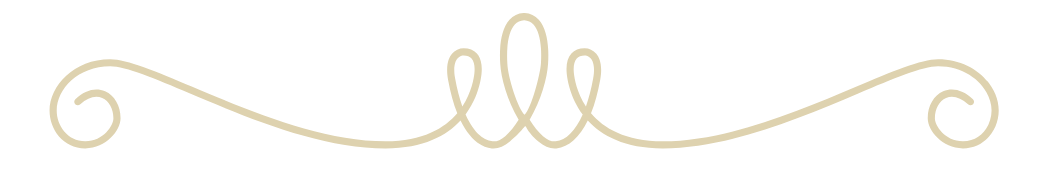/* styles */

## 2021 WINE EDUCATION SERIES WITH SWW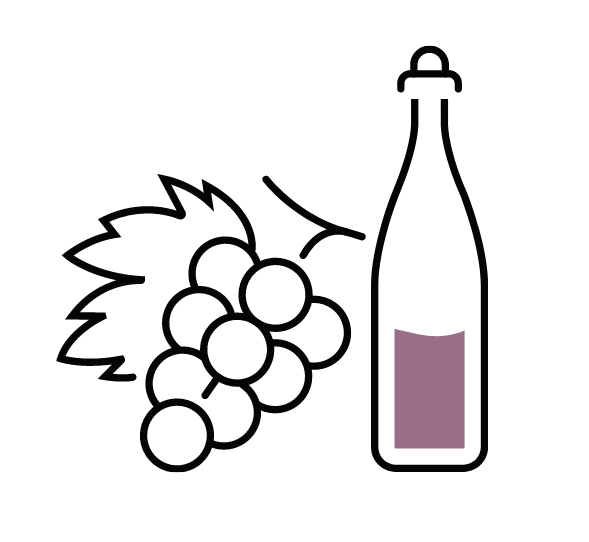Please join Regine T. Rousseau, Founder and CEO for an amazing line-up of educational events in 2021!

See the list below, and click to reserve your spot.

Want to level up your wine drinking style without the extra fluff? Shall We Wine is a resource that helps translate complex terminology into language that individuals of every level of wine & spirits proficiency can appreciate… all while having fun!

2021 SWW Fundamentals Class Series – our Fundamentals of Wine Series continues with a focus on helping you identify your wine style. The goal of these classes is to empower you to find the wines that fit your palate, the foods you enjoy and your mood. If you enjoy wine and are looking to deepen your knowledge of wine styles, grapes and regions, these are the classes for you!table div table+table+table+table+table+table+table+table+table+table+table+table div table{width:100%;padding:0}table div table+table+table+table+table+table+table+table+table+table+table+table div table img{width:96.23%;padding:0;float:none}table div table+table+table+table+table+table+table+table+table+table+table+table div table td{width:100%;padding:0 1.88% 18px}/* styles */## WELLNESS + SWWOur guest Mila Marshall kicked off January 2021 for Shall We Wine's Wellness Series. What a fabulous time to recharge! Participants learned about moving the breath with the body, understanding the healing benefits of the breath and were introduced to more breath practices to help destress and refocus.

The purpose of the Shall We Wine Wellness Series is to provide an outlet outside of the noise of the everyday, to focus on our wellness and well-being.

If you missed this Wellness Series event, don't fret! We have a few Wellness Series events sprinkled around for 2021.

→ Next up? Love After Covid with Dorian Nash!

 table div table+table+table+table+table+table+table+table+table+table+table+table+table+table+table div table{width:100%;padding:0}table div table+table+table+table+table+table+table+table+table+table+table+table+table+table+table div table img{width:96.23%;padding:0;float:none}table div table+table+table+table+table+table+table+table+table+table+table+table+table+table+table div table td{width:100%;padding:0 1.88% 18px}/* styles */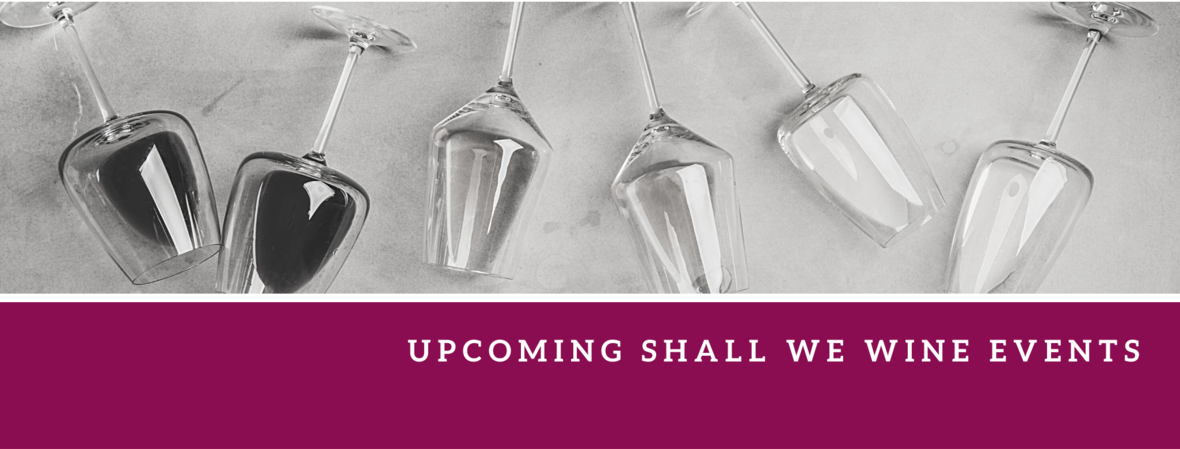/* styles */ Check out our newly updated events calendar to see what your next pour should be!table div table+table+table+table+table+table+table+table+table+table+table+table+table+table+table+table+table+table+table+table+table div table{width:100%;padding:0}table div table+table+table+table+table+table+table+table+table+table+table+table+table+table+table+table+table+table+table+table+table div table img{width:96.23%;padding:0;float:none}table div table+table+table+table+table+table+table+table+table+table+table+table+table+table+table+table+table+table+table+table+table div table td{width:100%;padding:0 1.88% 18px}/* styles */We are overjoyed to announce that Regine was selected as a recipient of the She Can Thrive 2020 Grant Program Award! What an honor!
_________
We are excited to partner with Govenor State University for a Fundementals of Food and Wine Class. Click here to enroll.

Class Description: Peas and carrots, peanut butter and jelly, steak and cabernet sauvignon...there is a synergy in our favorite food combinations. This same balance can be achieved with food and wine. We will teach you how to create unforgettable food and wine pairings at home, for events, and dining out. We will reveal some of the most cherished insider secrets and strategies for game changing food and wine synergy.

 table div table+table+table+table+table+table+table+table+table+table+table+table+table+table+table+table+table+table+table+table+table+table+table+table div table{width:100%;padding:0}table div table+table+table+table+table+table+table+table+table+table+table+table+table+table+table+table+table+table+table+table+table+table+table+table div table img{width:96.23%;padding:0;float:none}table div table+table+table+table+table+table+table+table+table+table+table+table+table+table+table+table+table+table+table+table+table+table+table+table div table td{width:100%;padding:0 1.88% 18px}/* styles */## HELPING ENTREPRENEURS SUCCEED/* styles */ Regine will be hosting the Accion Serving Illinois & Indiana Relaunch event! Join us virtually January 27th at 5:00 PM CST for a free event to hear from staff, partners, funders, and community businesses on how to work together to help more entrepreneurs build great businesses in 2021 and beyond. Click here for more information and to register!
 table div table+table+table+table+table+table+table+table+table+table+table+table+table+table+table+table+table+table+table+table+table+table+table+table+table+table+table+table div table{width:100%;padding:0}table div table+table+table+table+table+table+table+table+table+table+table+table+table+table+table+table+table+table+table+table+table+table+table+table+table+table+table+table div table img{width:96.23%;padding:0;float:none}table div table+table+table+table+table+table+table+table+table+table+table+table+table+table+table+table+table+table+table+table+table+table+table+table+table+table+table+table div table td{width:100%;padding:0 1.88% 18px}/* styles *//* styles */

## BLOG CENTRALWe have a blog! Here you will find things like our Wine of the Week and other special wine & spirits topics! Stay in the know and read up on some of the cool things we've been sharing.

 table div table+table+table+table+table+table+table+table+table+table+table+table+table+table+table+table+table+table+table+table+table+table+table+table+table+table+table+table+table+table+table+table div table{width:100%;padding:0}table div table+table+table+table+table+table+table+table+table+table+table+table+table+table+table+table+table+table+table+table+table+table+table+table+table+table+table+table+table+table+table+table div table img{width:96.23%;padding:0;float:none}table div table+table+table+table+table+table+table+table+table+table+table+table+table+table+table+table+table+table+table+table+table+table+table+table+table+table+table+table+table+table+table+table div table td{width:100%;padding:0 1.88% 18px}/* styles */## DRINK THIS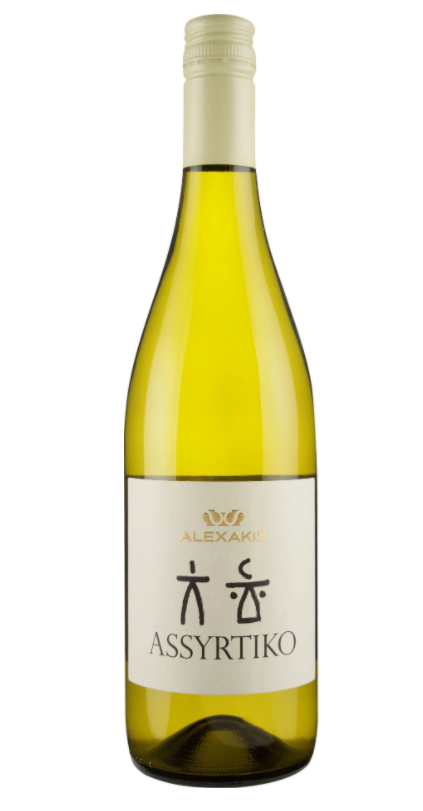Sometimes the hard part of venturing out of your comfortable wine bubble is simply figuring out where to start! We’re not in the biz of mandating excessive wine rules, and you should start wherever you feel comfortable. Don't let a wine bully convince you otherwise. (If you need a refresher on how to deal with a wine bully, read this!)

However, if you need a bit of a nudge, one way is to explore other regions you might not be as familiar with, or revisit ones you're not quite sure about.

There are fabulous wines produced in Greece, but people can often get intimidated by their overall lack of familiarity with them or even more simply, how to pronounce the grape names! But you can get some definite bang-for-your-buck wines from Greece.

Today we highlight an Assyrtiko (pronounced ah-seer-ti-ko) from Crete: Alexakis Assyrtiko! Assyrtiko, in general, is a remarkable, versatile white wine from Greece, originating from the island of Santorini. Alexakis Assyrtiko in particular, is a refreshing take on the prized grape. It is crisp, with a comforting lemon cream feel, without an overpowering backbone. Easily paired with shellfish!

 table div table+table+table+table+table+table+table+table+table+table+table+table+table+table+table+table+table+table+table+table+table+table+table+table+table+table+table+table+table+table+table+table+table+table+table div table{width:100%;padding:0}table div table+table+table+table+table+table+table+table+table+table+table+table+table+table+table+table+table+table+table+table+table+table+table+table+table+table+table+table+table+table+table+table+table+table+table div table img{width:96.23%;padding:0;float:none}table div table+table+table+table+table+table+table+table+table+table+table+table+table+table+table+table+table+table+table+table+table+table+table+table+table+table+table+table+table+table+table+table+table+table+table div table td{width:100%;padding:0 1.88% 18px}/* styles */## ENGAGING READS FOR THE MONTHtable div table+table+table+table+table+table+table+table+table+table+table+table+table+table+table+table+table+table+table+table+table+table+table+table+table+table+table+table+table+table+table+table+table+table+table+table+table+table div table{width:100%;padding:0}table div table+table+table+table+table+table+table+table+table+table+table+table+table+table+table+table+table+table+table+table+table+table+table+table+table+table+table+table+table+table+table+table+table+table+table+table+table+table div table img{width:96.23%;padding:0;float:none}table div table+table+table+table+table+table+table+table+table+table+table+table+table+table+table+table+table+table+table+table+table+table+table+table+table+table+table+table+table+table+table+table+table+table+table+table+table+table div table td{width:100%;padding:0 1.88% 18px}/* styles */## STAY CONNECTED

 /* styles */ To stay in the know with the latest and greatest, make sure to follow us! Links are below.
 table div table+table+table+table+table+table+table+table+table+table+table+table+table+table+table+table+table+table+table+table+table+table+table+table+table+table+table+table+table+table+table+table+table+table+table+table+table+table+table+table+table div table{width:100%;padding:0}table div table+table+table+table+table+table+table+table+table+table+table+table+table+table+table+table+table+table+table+table+table+table+table+table+table+table+table+table+table+table+table+table+table+table+table+table+table+table+table+table+table div table table{padding:0;float:left!important;width:26.059%!important}table div table+table+table+table+table+table+table+table+table+table+table+table+table+table+table+table+table+table+table+table+table+table+table+table+table+table+table+table+table+table+table+table+table+table+table+table+table+table+table+table+table div table td{padding-left:29px;padding-right:29px}table div table+table+table+table+table+table+table+table+table+table+table+table+table+table+table+table+table+table+table+table+table+table+table+table+table+table+table+table+table+table+table+table+table+table+table+table+table+table+table+table+table div table table+table,table div table+table+table+table+table+table+table+table+table+table+table+table+table+table+table+table+table+table+table+table+table+table+table+table+table+table+table+table+table+table+table+table+table+table+table+table+table+table+table+table+table div table table+table+table{float:left!important;width:26.059%!important}table div table+table+table+table+table+table+table+table+table+table+table+table+table+table+table+table+table+table+table+table+table+table+table+table+table+table+table+table+table+table+table+table+table+table+table+table+table+table+table+table+table div table table td,table div table+table+table+table+table+table+table+table+table+table+table+table+table+table+table+table+table+table+table+table+table+table+table+table+table+table+table+table+table+table+table+table+table+table+table+table+table+table+table+table+table div table table+table td,table div table+table+table+table+table+table+table+table+table+table+table+table+table+table+table+table+table+table+table+table+table+table+table+table+table+table+table+table+table+table+table+table+table+table+table+table+table+table+table+table+table div table table+table+table td{padding-left:0;padding-right:20px}table div table+table+table+table+table+table+table+table+table+table+table+table+table+table+table+table+table+table+table+table+table+table+table+table+table+table+table+table+table+table+table+table+table+table+table+table+table+table+table+table+table div table table+table+table+table{float:left!important;width:21.822999999999993%!important}table div table+table+table+table+table+table+table+table+table+table+table+table+table+table+table+table+table+table+table+table+table+table+table+table+table+table+table+table+table+table+table+table+table+table+table+table+table+table+table+table+table div table table+table+table+table td{padding-left:0;padding-right:0}/* styles */table div table+table+table+table+table+table+table+table+table+table+table+table+table+table+table+table+table+table+table+table+table+table+table+table+table+table+table+table+table+table+table+table+table+table+table+table+table+table+table+table+table+table+table div table{width:100%;padding:0}table div table+table+table+table+table+table+table+table+table+table+table+table+table+table+table+table+table+table+table+table+table+table+table+table+table+table+table+table+table+table+table+table+table+table+table+table+table+table+table+table+table+table+table div table img{width:96.23%;padding:0;float:none}table div table+table+table+table+table+table+table+table+table+table+table+table+table+table+table+table+table+table+table+table+table+table+table+table+table+table+table+table+table+table+table+table+table+table+table+table+table+table+table+table+table+table+table div table td{width:100%;padding:0 1.88% 18px}/* styles */## (VIRTUAL) HUGS FROM USIn an effort to maintain social distancing measures, Shall We Wine will continue to devote our time to connecting with each and every one of our friends and supporters like you virtually. We're constantly sharing fun content to help brighten up your weeks!

THANK YOU for supporting this small business. We love you and will continue to champion for the excitement and enthusiasm you have for the beverage experience ❤

We appreciate your patience and cooperation as we work through these times together. Thank you for your continued support in making Shall We Wine your go-to wine & lifestyle resource!/* styles */ Learn, Drink, Repeat! The Shall We Wine Team
 Like   Tweet   in